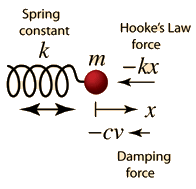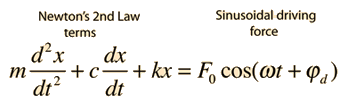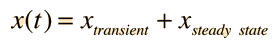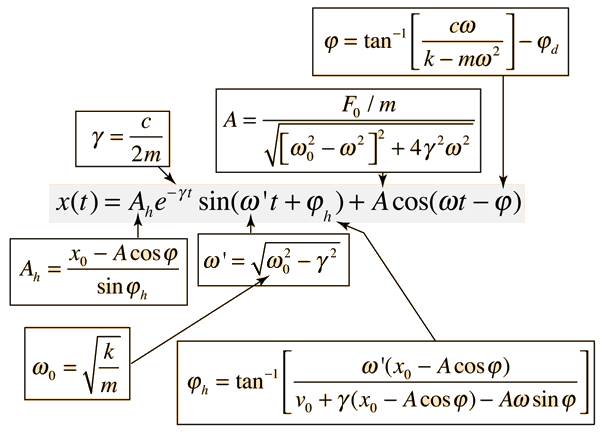# Driven Oscillator

If a damped oscillator is driven by an external force, the solution to the motion equation has two parts, a transient part and a steady-state part, which must be used together to fit the physical boundary conditions of the problem.The motion equation is of the formand has a general solutionIn the underdamped case this solution takes the formThe initial behavior of a damped, driven oscillator can be quite complex. The parameters in the above solution depend upon the initial conditions and the nature of the driving force, but deriving the detailed form is an involved algebra problem. The form of the parameters is shown below.

### Examples of driven oscillators

 Transient solution Steady-state solution Expansion of above terms
Index

Periodic motion concepts

 HyperPhysics***** Mechanics R Nave
Go Back

# Underdamped Driven Oscillator

The expanded expressions for the underdamped oscillator in terms of the mass, spring constant, damping, and driving force. They are also affected by the initial values of position and velocity.Index

Periodic motion concepts

Reference

Barger & Olsson

Marion & Thornton, Ch 3

 HyperPhysics***** Mechanics R Nave
Go Back

# Transient Solution, Driven Oscillator

The solution to the driven harmonic oscillator has a transient and a steady-state part. The transient solution is the solution to the homogeneous differential equation of motion which has been combined with the particular solution and forced to fit the physical boundary conditions of the problem at hand. The form of this transient solution is that of the undriven damped oscillator and as such can be underdamped, overdamped, or critically damped.

### Examples of driven oscillators

Index

Periodic motion concepts

 HyperPhysics***** Mechanics R Nave
Go Back